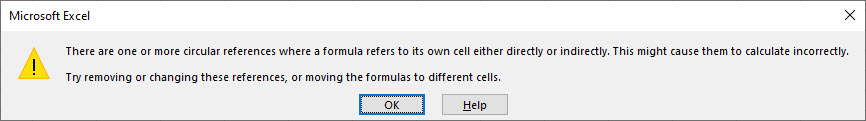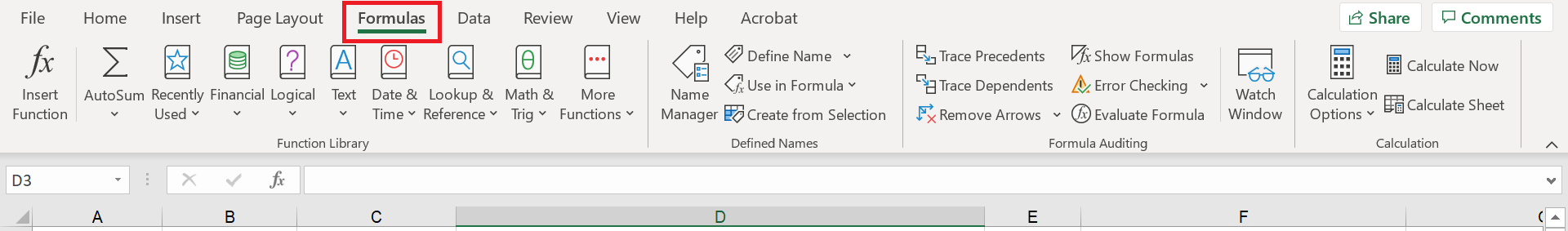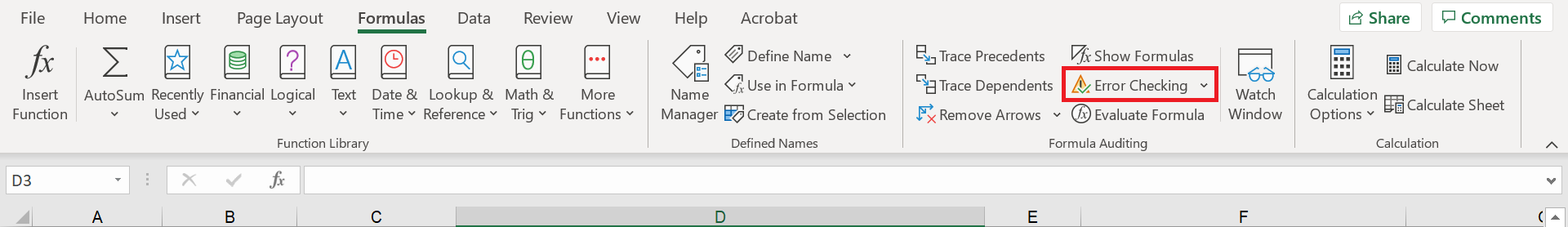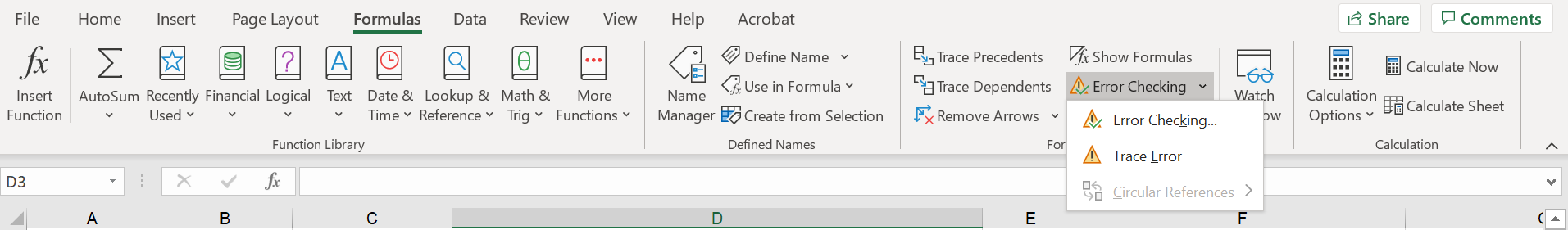#### The days where accounting and finance professionals have manual and time-consuming processes should be a thing of the past. The majority of finance professionals today will have Microsoft Excel in their personal toolbox, but are they using it to the best of its abilities?

Circular references are one of those things most people have heard of, but not many fully understand. We are here to tell you why you should not be using circular references and what you should be doing instead.

But first, what is a circular reference?

Simply put, a circular reference occurs when an Excel formula refers to its own cell, either directly or indirectly, often for forward-looking calculations such as interest and tax.

The simplest example would be if in cell A1 you typed in “=A1”, this would be a circular reference as this formula refers to its own result. Most circularities involve a chain of references and can be quite difficult to follow. In any case Excel will alert you as shown below:Why should you avoid circular references?

There are many reasons we advise against using circular references as we’ll discuss below, but a key one is that in many cases, the result can be calculated algebraically. This will be discussed in more detail when looking at the alternatives to circular references.

It is possible, in many cases, to resolve circular references by switching on iterative calculations, but we do not recommend this. Iterative calculations are, as the name suggests, when circular calculations in a workbook are recalculated until a specific number of iterations is met i.e. complete the calculation 10 times. The issues with turning iterative calculations on are as follows:

• Switching iterative calculations on is at the application level, not the workbook level, which will impact other workbooks. Ultimately, this means that iterative calculations will be applied to every open workbook, not just the workbooks that have circular references.

• Excel will no longer warn you if you introduce another circular reference. This makes it possible to accidentally create new circular references and difficult to track them down and resolve them.

• Resolving a circularity by using iterative calculation relies on it being a convergent one – this is not always the case and it is possible to get an Excel file that doesn’t resolve on a stable answer. This would mean that every time you re-calculate the Excel file (click F9 to do this manually), you would get a different result.

• If you have iterative calculations turned on, and then close and re-open Excel, iterative calculations will be turned off. This means that every time you open Excel back up, you will need to go into the settings and turn iterative calculations back on in order for your circular workbook to calculate correctly. If you have a second workbook open when you close your intentionally circular one, iterative calculation will remain switched on.

• A final smaller issue with circular references is that Excel will give you a warning message every time a workbook is opened that has a circular reference (unless you already have iterative calculations on), which, if nothing else, can be an irritation.

So, why are circular references used?

Circular references are used for a variety of reasons, but the most common reason is that they are accidentally created by users who do not really understand their impact. An example we see is a ‘fat finger’ when using the SUM function, such as typing in cell A1, “=SUM(A1:B2)”. This is very easily done by even the most experienced Excel users. On top of this, if you do not know what a circular reference is, the error message that pops up when creating one can be easily dismissed and be assumed to not impact your workbook.

When completing interest and tax calculations circular references are also used. The reason for this is that these calculations tend to have forward-looking logic (which is inherently circular in nature), such as calculating in-year tax payments based on the full-year tax charge.

Another example of an inherently circular calculation is sculpting debt based on a Debt Service Coverage Ratio (DSCR). This is a technique used to tailor the debt repayments to the pattern of the cash flows of the project, often used in project finance models. If you’re interested in finding out more, we have covered the topic of the DSCR in another blog here.

How do you find circular references?

In order to eliminate circular references, you first need to identify all existing circular references in your workbook:

Step 1 – Go the ribbon and click on “Formulas”Step 2 – Click on the down arrow next to “Error Checking”Step 3 – Hover over “Circular References” and it will show you all of the circular references that Excel is registering currently (you can see in the example that there is only one circular reference in cell A1). What I mean by “registering currently” is that there may be more circular references in the workbook than the shown circular references, and the only way to find that out is to remove the ones currently shown in that list, and then go back and see if anymore have appeared in the list.What should you use instead of circular references?

Many inherently circular calculations, such as forward-looking calculations can be resolved algebraically, eliminating the need for a circular reference. It may just need a little more time invested trying to figure it out, but the end result is much more efficient and reliable.

In some instances, the value being calculated by a circular reference is immaterial and the spurious accuracy gained by the circular reference (with all its inherent risks) is outweighed by the uncertainty in all the other input assumptions. Where there is an inherently circular calculation that can’t be resolved algebraically, it can be controlled with a simple VBA macro to ‘break the circularity’ and avoid the risk. We categorise these macros into two types:

• Copy and paste – this essentially makes the circular part of the calculation a hard-coded value i.e. it is not a formula anymore and so cannot be circular.

• Goal seek – this also uses hard-coded inputs, but uses the Goal Seek functionality that is built into excel to reduce the difference between the input and the output to close to zero. We often initiate Goal Seek with a macro rather than clicking a button in the ribbon (Data>What-If Analysis>Goal Seek). Using VBA allows for automation and more flexibility when ‘goal-seeking’.

A final alternative to using circular references is to just simplify the calculation. In our experience the value being calculated by a circular reference will be immaterially different than a simpler non-circular calculation, and for the sake of all of the risks and issues that circular references bring, why not simplify the calculation?

#### Key Takeaways

We have discussed circular references in detail in this article, covering off the key reasons to avoid them, how to resolve them and what you should be using instead.

Here are the key takeaways summarised for you:

• We know that the majority of modern-day finance professionals are using Excel, but not to the best of its abilities.

• A circular reference occurs when an Excel formula refers to its own cell, either directly or indirectly.

• The most common creation of circular references is by accident, closely followed by forward-looking calculations, such as interest and tax calculations.

• The first step to ridding yourself of circular references is to know how to identify them.

• The main alternative to using a circular reference is an algebraic solution, or to use VBA.